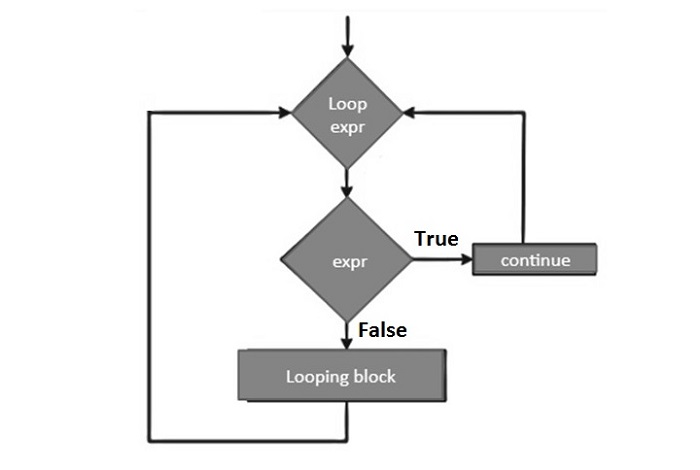# Python - The Continue Statement

The continue statement in Python returns the control to the beginning of the current loop. When encountered, the loop starts next iteration without executing the remaining statements in the current iteration.

The continue statement can be used in both while and for loops.

### Syntax

```continue
```

### Flow Diagram

The flow diagram of the continue statement looks like this −The continue statement is just the opposite to that of break. It skips the remaining statements in the current loop and starts the next iteration.

### Example 1

Now let's take an example to understand how the continue statement works in Python −

```for letter in 'Python': # First Example
if letter == 'h':
continue
print ('Current Letter :', letter)
var = 10 # Second Example
while var > 0:
var = var -1
if var == 5:
continue
print ('Current variable value :', var)
print ("Good bye!")
```

When the above code is executed, it produces the following output

```Current Letter : P
Current Letter : y
Current Letter : t
Current Letter : o
Current Letter : n
Current variable value : 9
Current variable value : 8
Current variable value : 7
Current variable value : 6
Current variable value : 4
Current variable value : 3
Current variable value : 2
Current variable value : 1
Current variable value : 0
Good bye!
```

### Example 2: Checking Prime Factors

Following code uses continue to find the prime factors of a given number. To find prime factors, we need to successively divide the given number starting with 2, increment the divisior and continue the same process till the input reduces to 1.

The algorithm for finding prime factors is as follows −

• Accept input from user (n)

• Set divisor (d) to 2

• Perform following till n>1

• Check if given number (n) is divisible by divisor (d).

• If n%d==0

• a. Print d as a factor

• Set new value of n as n/d

• Repeat from 4

• If not

• Increment d by 1

• Repeat from 3

Given below is the Python code for the purpose −

```num = 60
print ("Prime factors for: ", num)
d=2
while num>1:
if num%d==0:
print (d)
num=num/d
continue
d=d+1
```

On executing, this code will produce the following output

```Prime factors for: 60
2
2
3
5
```

Assign different value (say 75) to num in the above program and test the result for its prime factors.

```Prime factors for: 75
3
5
5
```